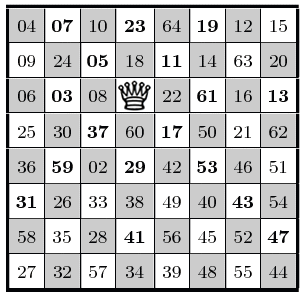# 499

This number is a prime.The smallest prime which is the sum of a prime number (pn) of first odd primes. Note that n is itself the pmth odd prime, where m is the smallest prime. [Beedassy]The decimal expansion of 499499 ends with the digits 499499. (Often pp ends with the digits of p, but this is the only known case for which it ends with the digits of p twice.) [Caldwell]Separate 499 into two (49, 9) and three groups (4, 9, 9) and get two primes after replacing each subset with its square root, namely 73 and 233. Replacing each digit of these latter two primes with their cubes results also in two new primes 34327 and 82727. [De Geest]The distance light travels the vacuum of space in 499 seconds is approximately the mean distance between the centers of the Earth and Sun (an Astronomical Unit).499 = 497 + 2 is the reversal of 497 * 2.The largest prime Köchel number of Mozart's string quartets (String Quartet No. 20 in D). [Hasler]A Knight's Tour
A knight's tour is a numbered tour of a knight over an otherwise empty chessboard visiting each square once only. A queen placed on the start of a tour discovered by George Jelliss can attack all of the odd primes, and every odd number attacked by the queen is a prime. It is also a more restricted version known as a re-entrant tour, in which the knight, on its 64th move, could arrive back at its starting square. The sum of the odd primes in the tour is 499.The largest 3-digit composite-digit prime. [Loungrides]The year Hindu mathematician Aryabhata (ar-yuh-BUHT-uh) published a famous book on mathematics and astronomy in verse.Grosswald (1981) proved that if a prime p is greater than ee24 then the smallest primitive root of p is less than p0.499. [Gudipati]The Scramble Squares Puzzle has 4^9*9! different arrangements of the nine pieces. [Hale]The smallest prime of the form 5*10^n-1. [Shepherd]The viewpoint hike at Chimney Rock State Park in North Carolina requires 499 steps.499 is the sum of an nth perfect number + n (case n = 3). Is there a greater example? [Worrom]Sum of digits of all primes up to 499 is the smallest bemirp. [Sariyar]

(There are 7 curios for this number that have not yet been approved by an editor.)